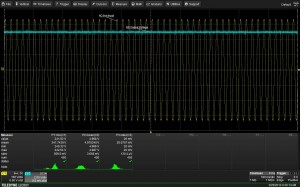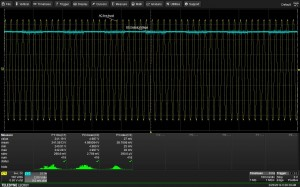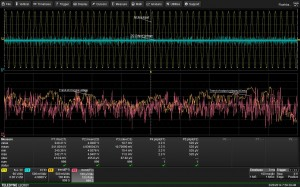• +44 (0)1793 784389

# Power Line Monitoring and Data Logging

## Using the trend function for monitoring long term processes

The trend function is an ideal data logging tool, for applications like power line monitoring, taking place over seconds, minutes, hours, or days.  It is a waveform composed of a series of parameter measurement values presented in the order they were taken. Up to 1,000 values can be trended. This is an ideal tool for monitoring long term processes such as power line monitoring. Figure 1 shows a power supply test where the input line voltage and output voltage are monitored.Figure 1: An example of measuring the AC line input and DC output of a power supply using parameters and statistics

The AC line input is acquired on channel 1 (top yellow trace) using a differential probe.  Channel 3 (blue trace) is the DC output of the power supply. Record length for each measurement is one second. The parameter P1 is the rms value of the power line. Parameter P2 is the mean value of the DC output voltage. Parameter P3 is the standard deviation (ACrms) value of the power supplies output. The statistics are used to measure changes for each parameter. The parameter P3, for example, is used to monitor small changes in the power supply output level including ripple and line related output changes.

In the figure 2, the input line and the output voltage are monitored using the trend functions during a long acquisition (17 min).Figure 2: An example of measuring the AC line input and DC output of a power supply using trend function

Function trace F1 is a trend of the AC input voltage. It shows the rms line voltage over 1000 acquisitions (about 17 minutes). Similarly, the function trace F2 shows the trend of P3 (standard deviation) taken at the same time. The trend of line voltage shows variations on the order of 2.3 V over the 17 minute interval as read by P4 (peak-to-peak of F1). At the same time the AC variation of the power supply output is 535 μV. The mean power supply output (P2) is 4.939V.

Trend functions can be set up from the Math setup dialog box as shown in Figure 3 below.Figure 3: Setting up a trend function using the math setup dialog box for function F1

The source of the trend can be any parameter. The number of values (points) in a trend can range from 2 to 1,000 . Scaling can be set automatically or manually. It is important to note that the trend function can be manipulated like any waveform, math operations and parameter measurements can be applied.

We also can compute the line variation using the measurements taken thus far.

### Conclusion

The trend function of an oscilloscope is incredibly useful to log data over time periods from a few seconds to several days, particularly in applications such as power line monitoring.